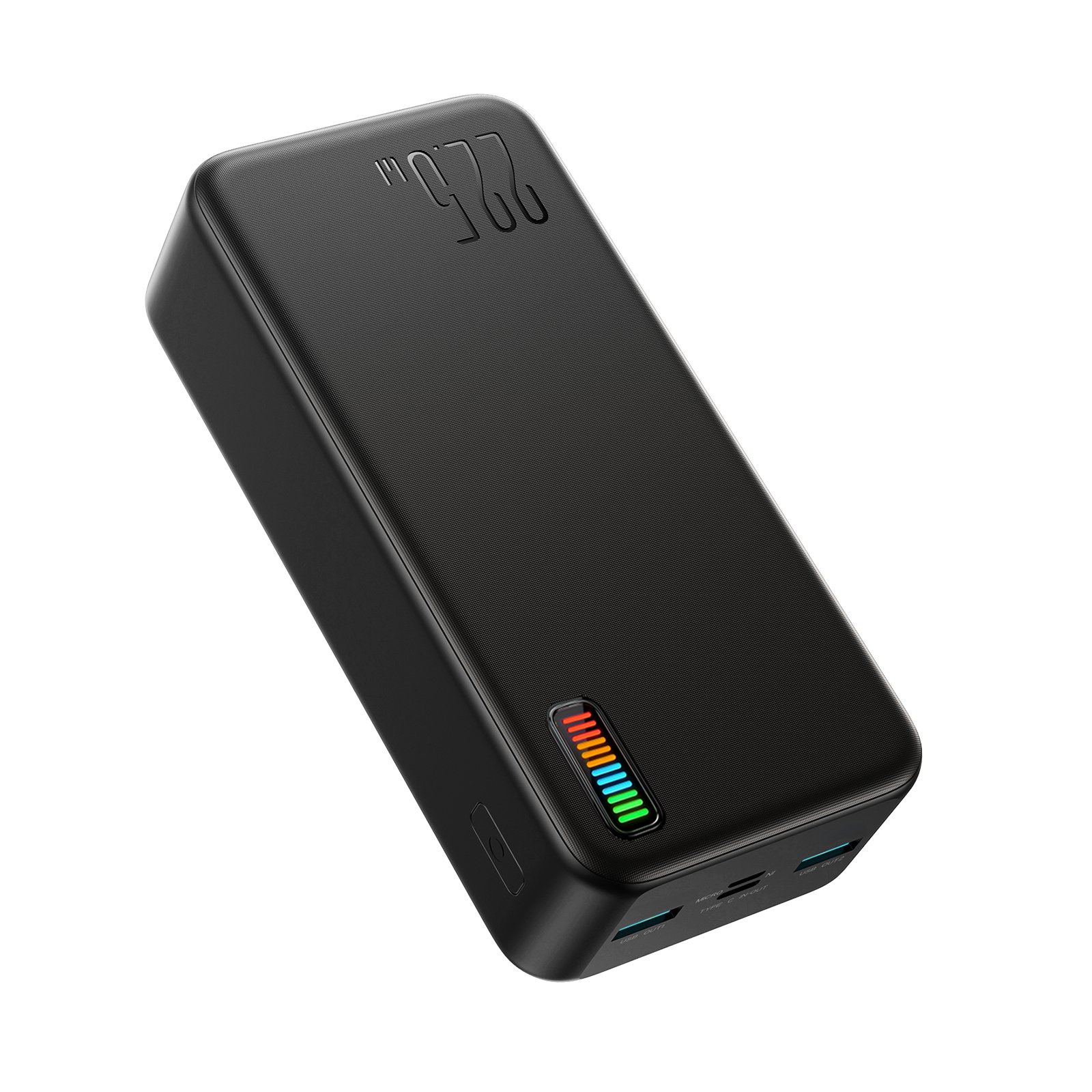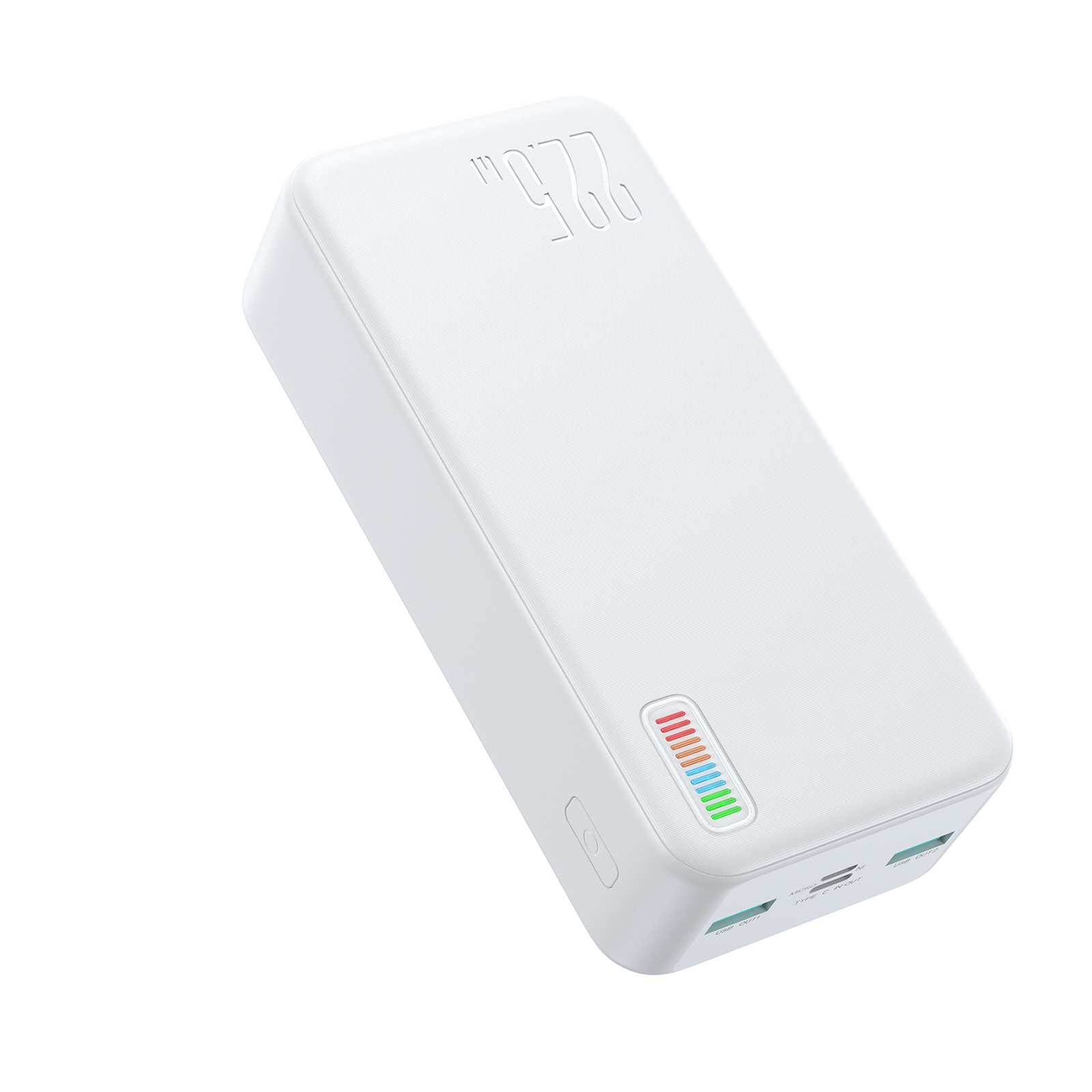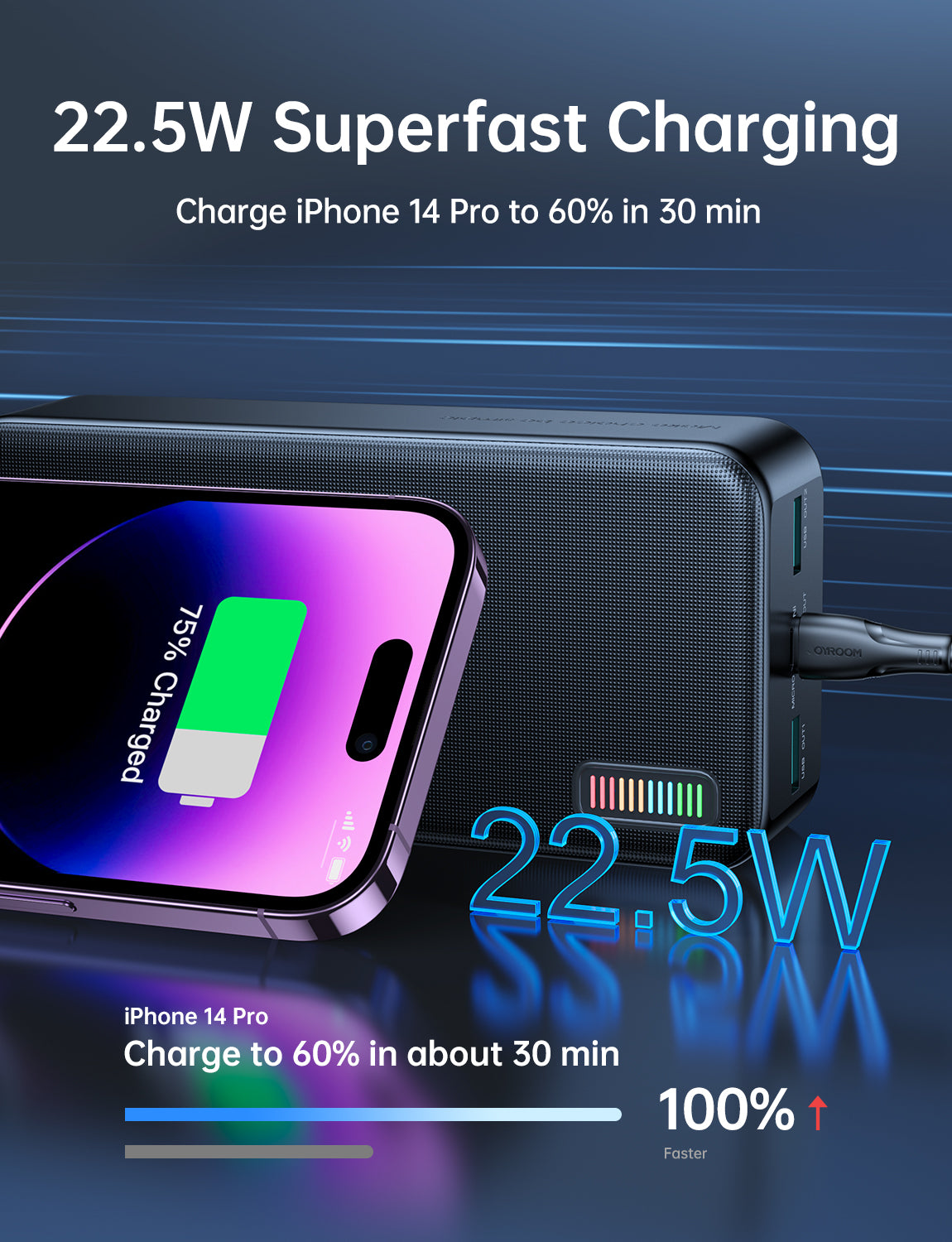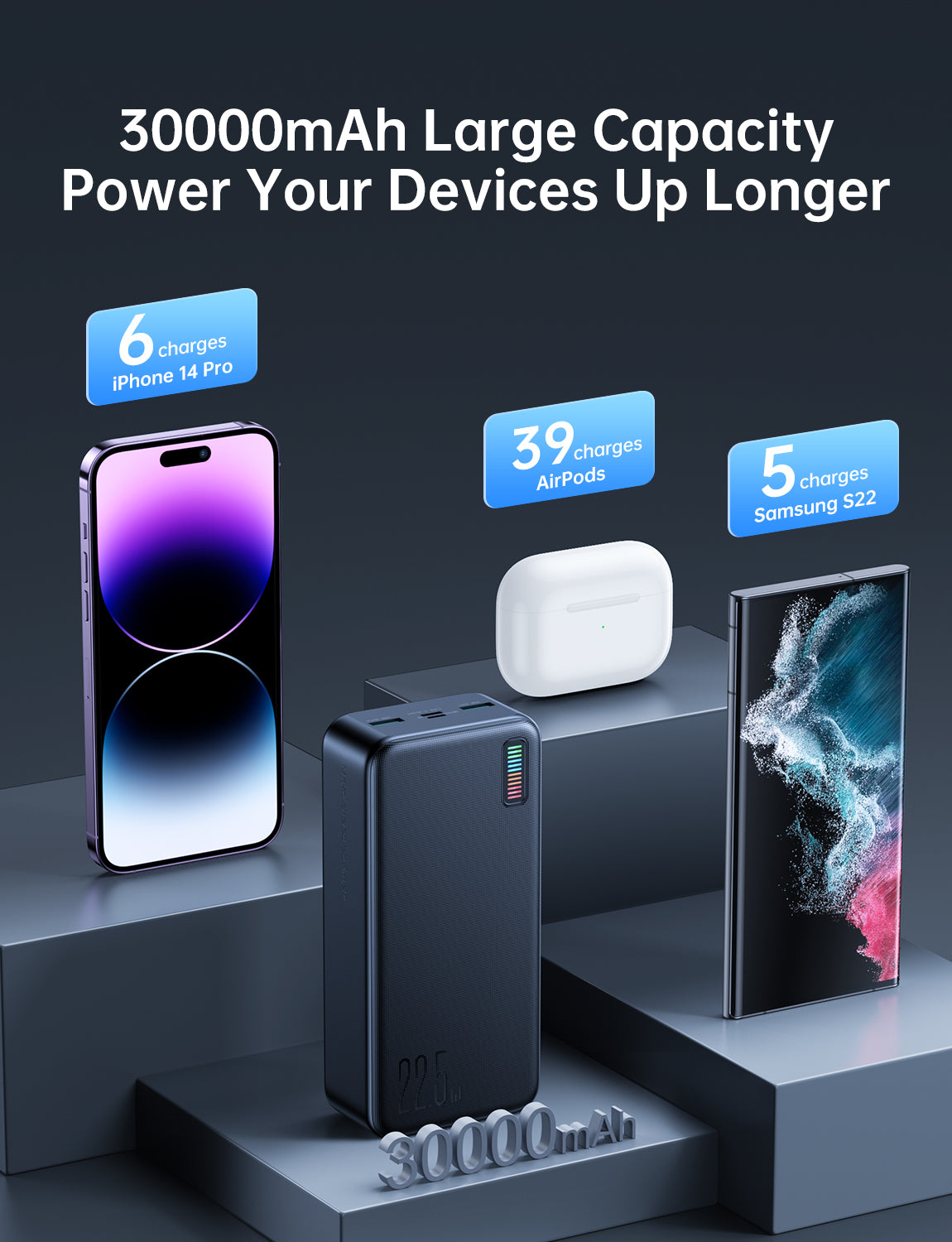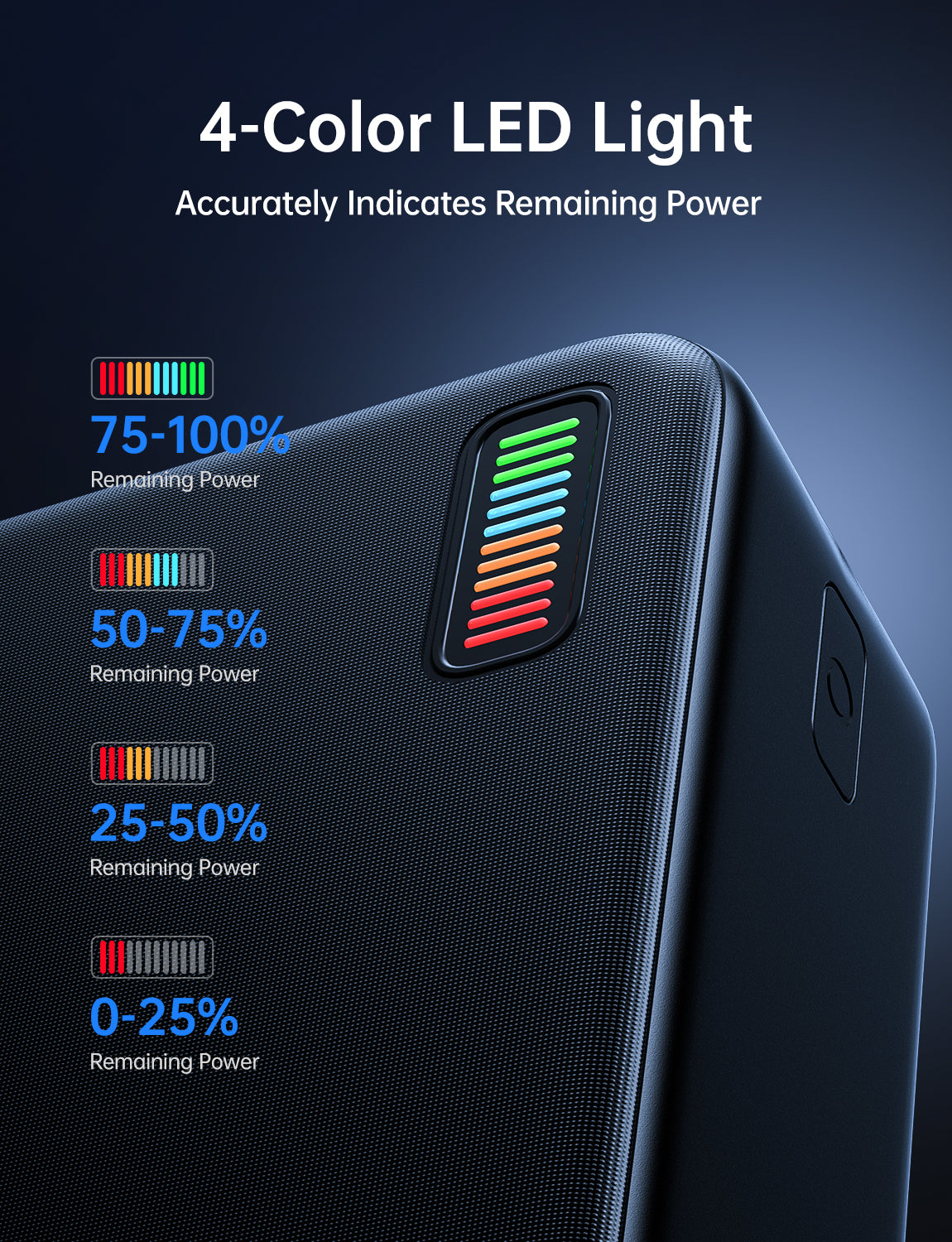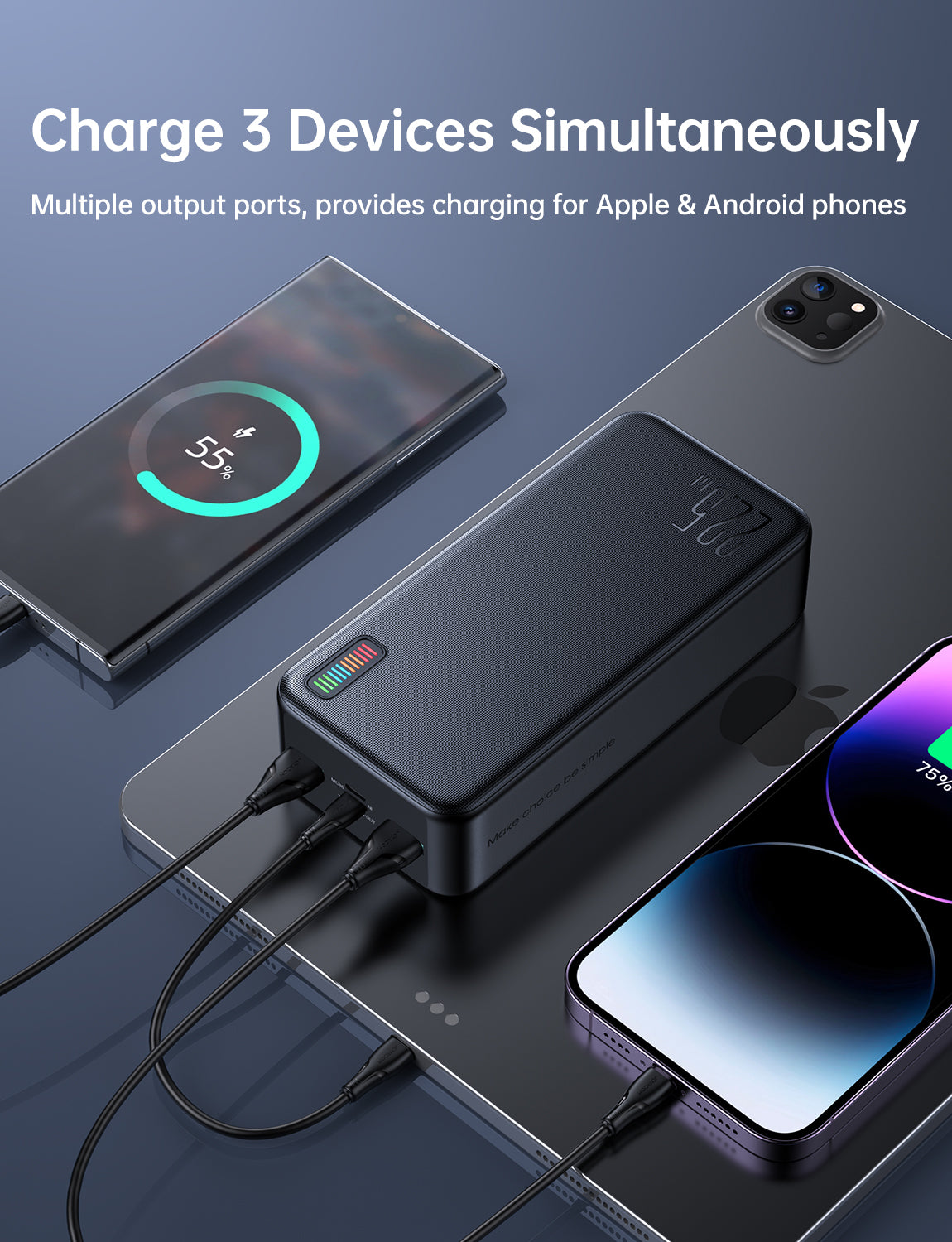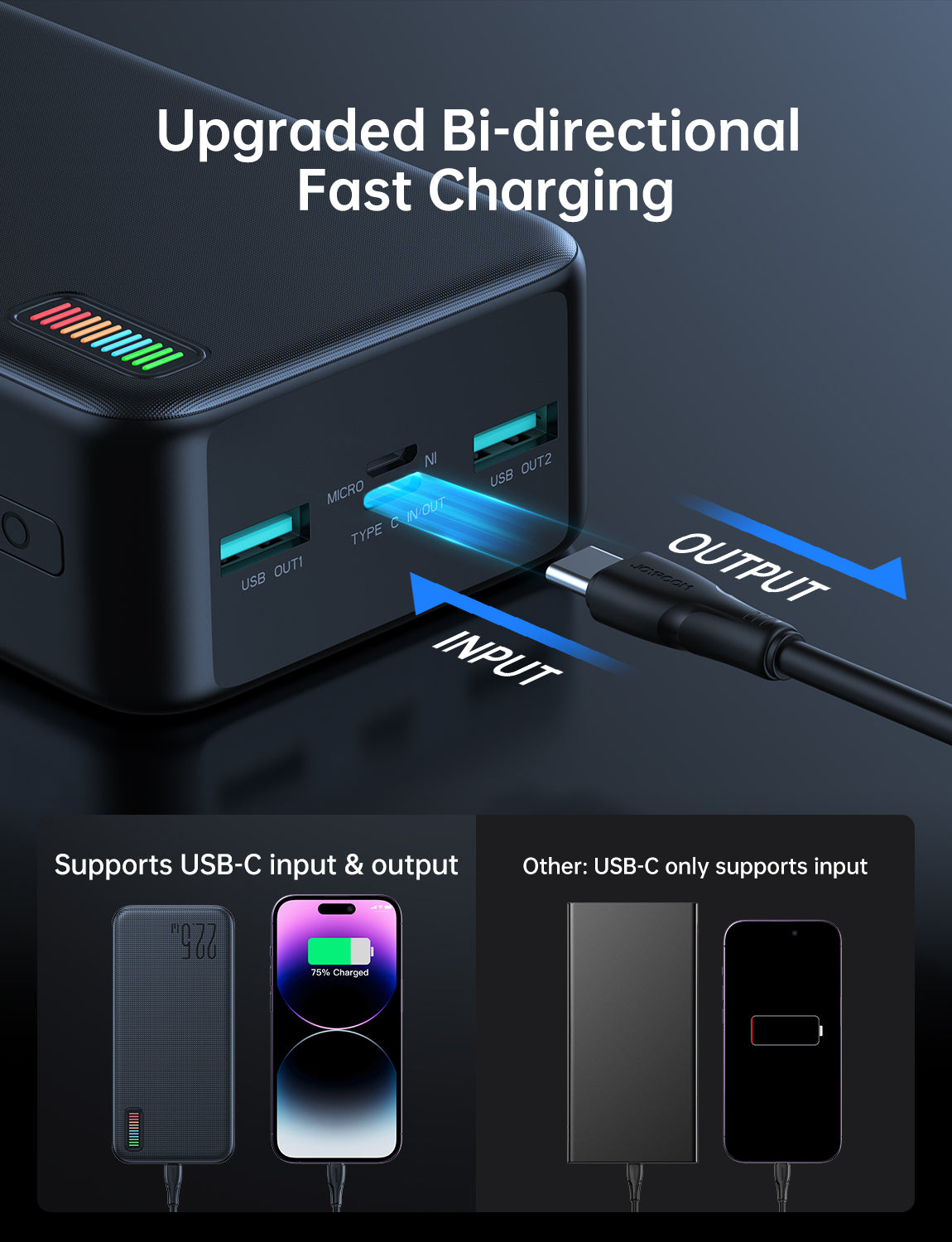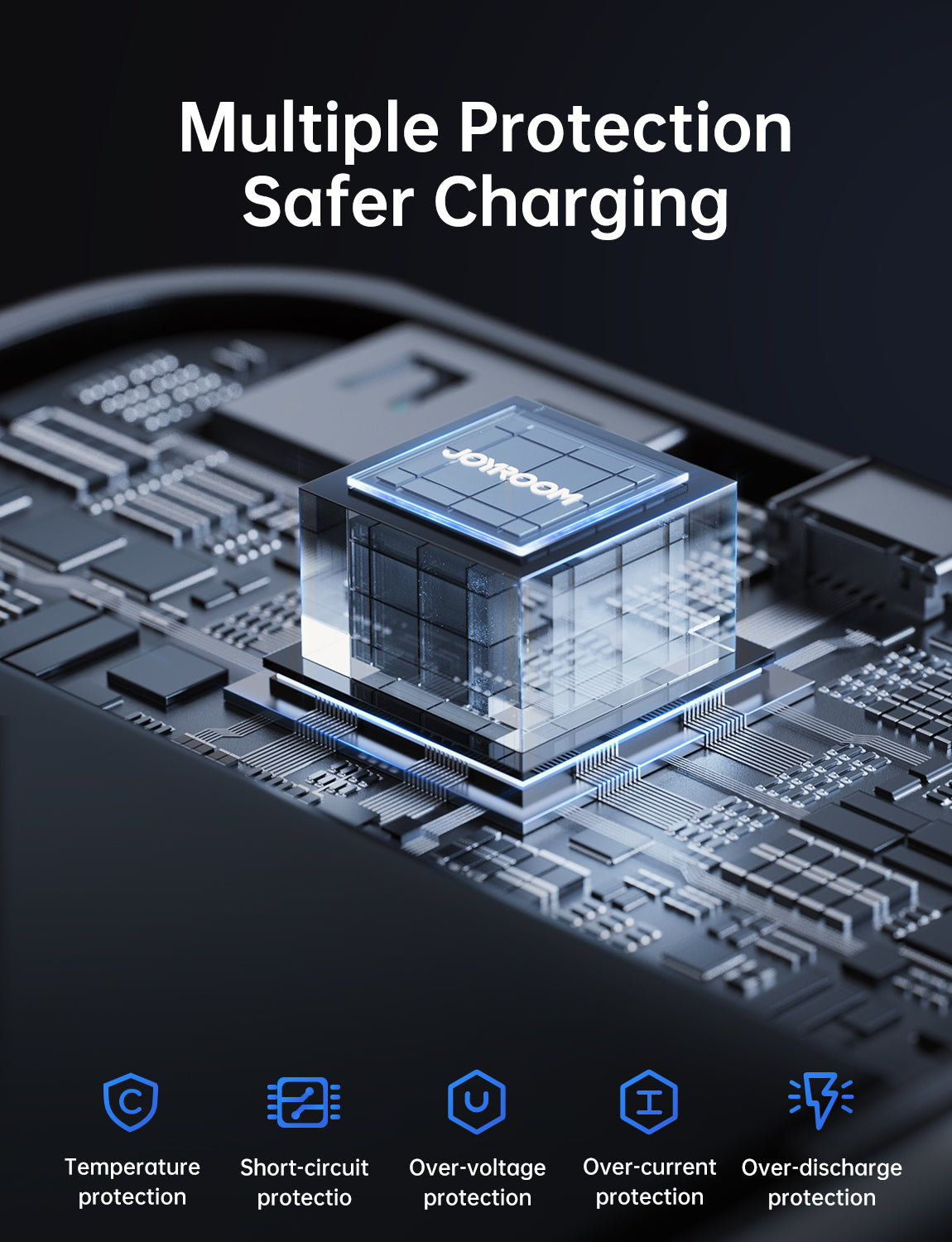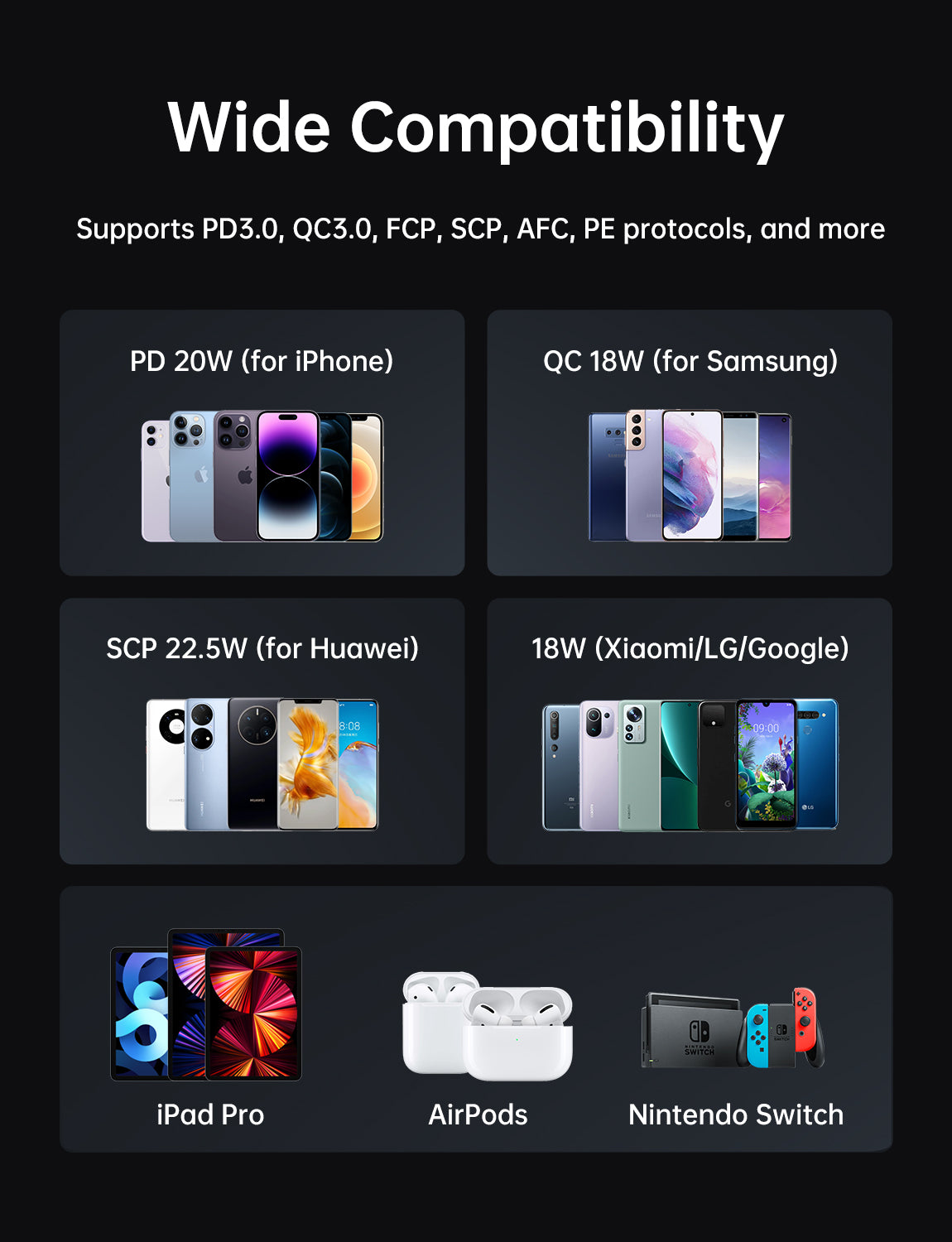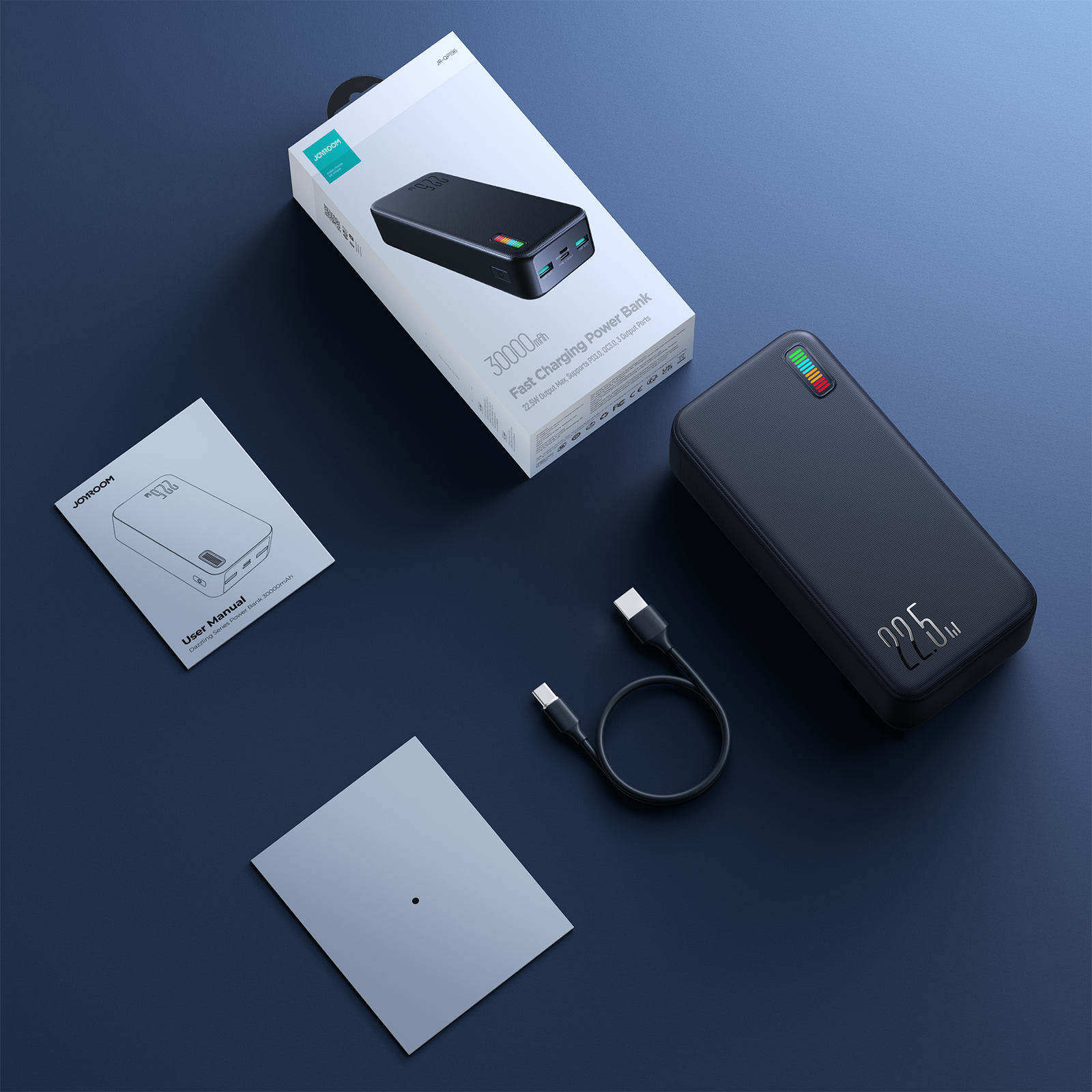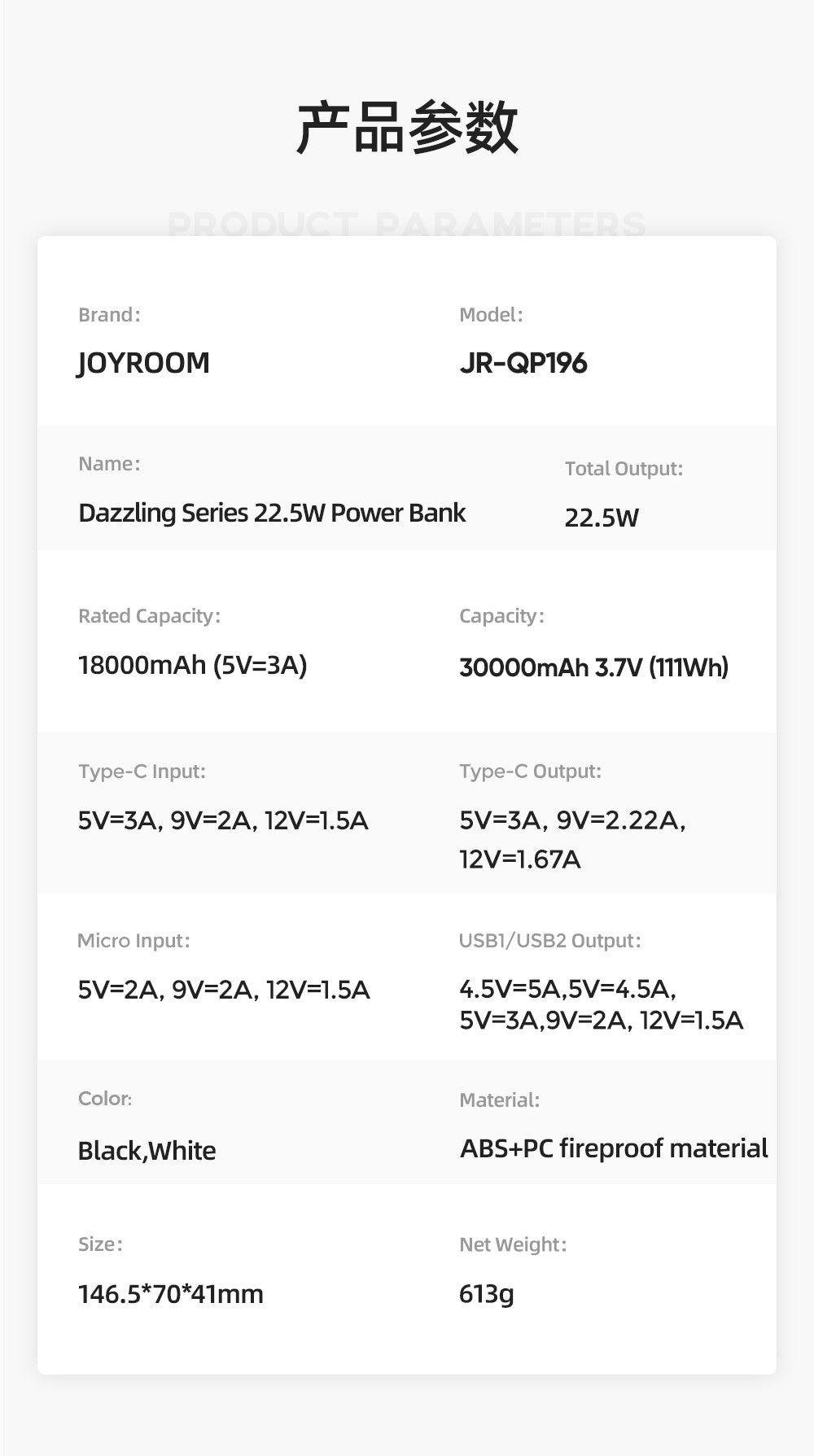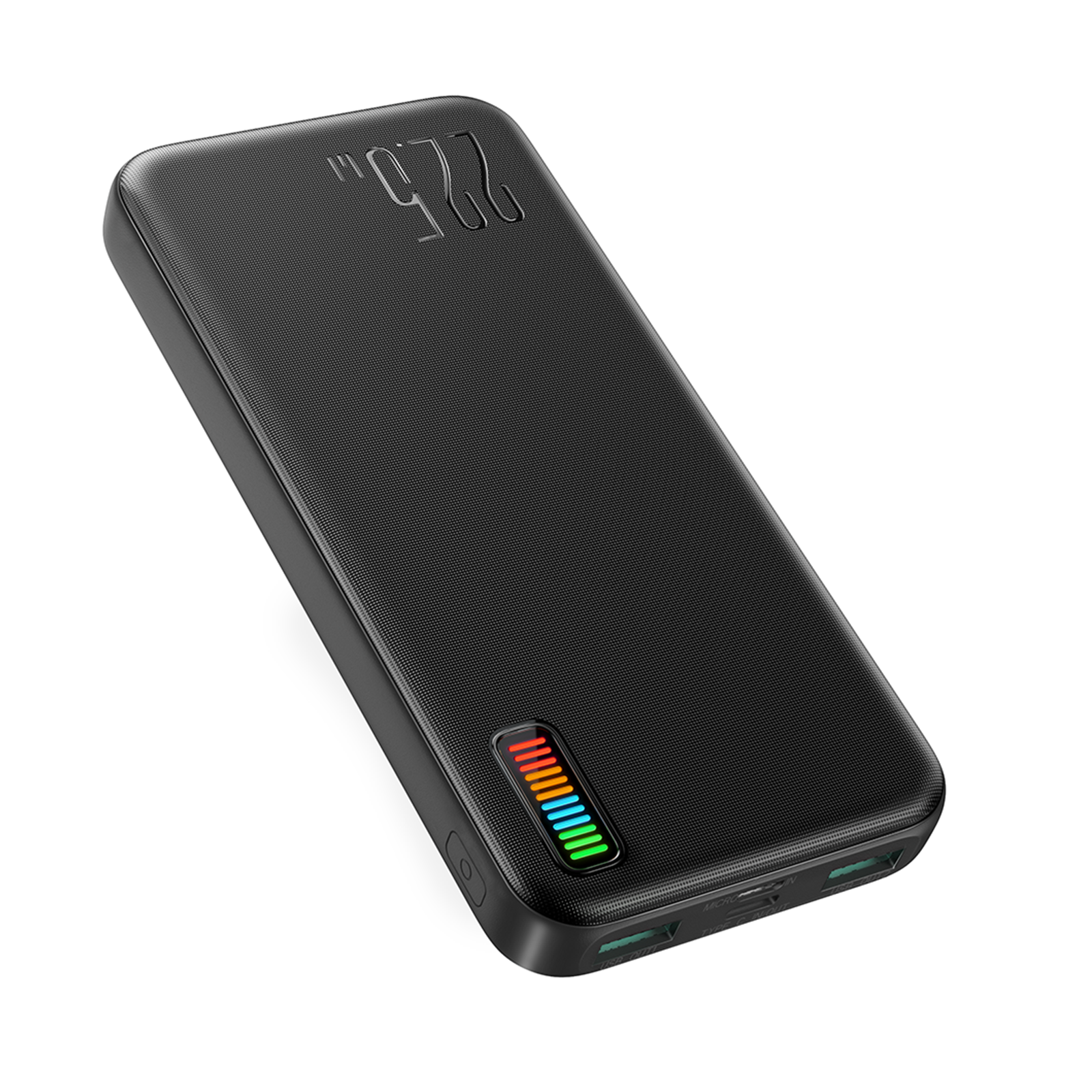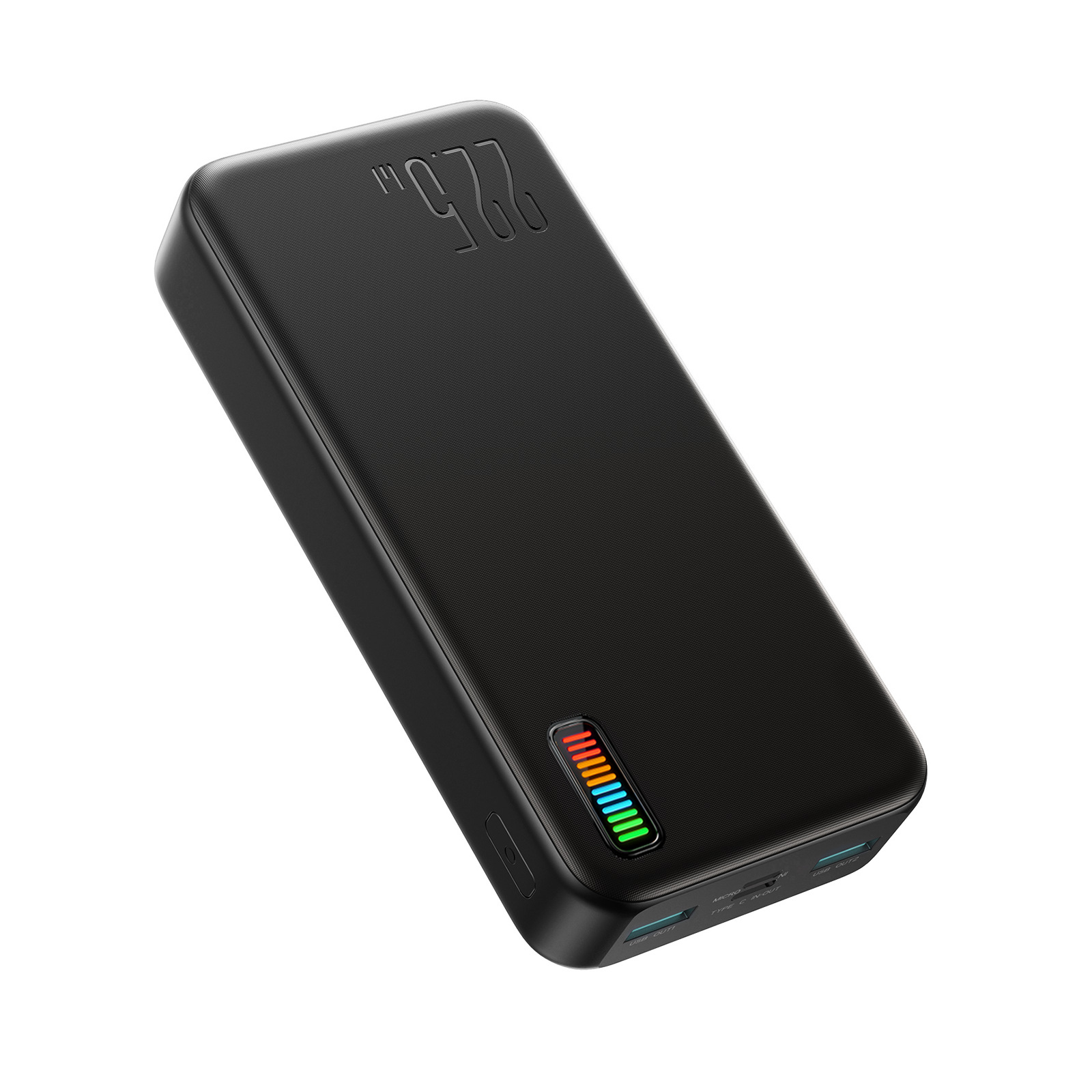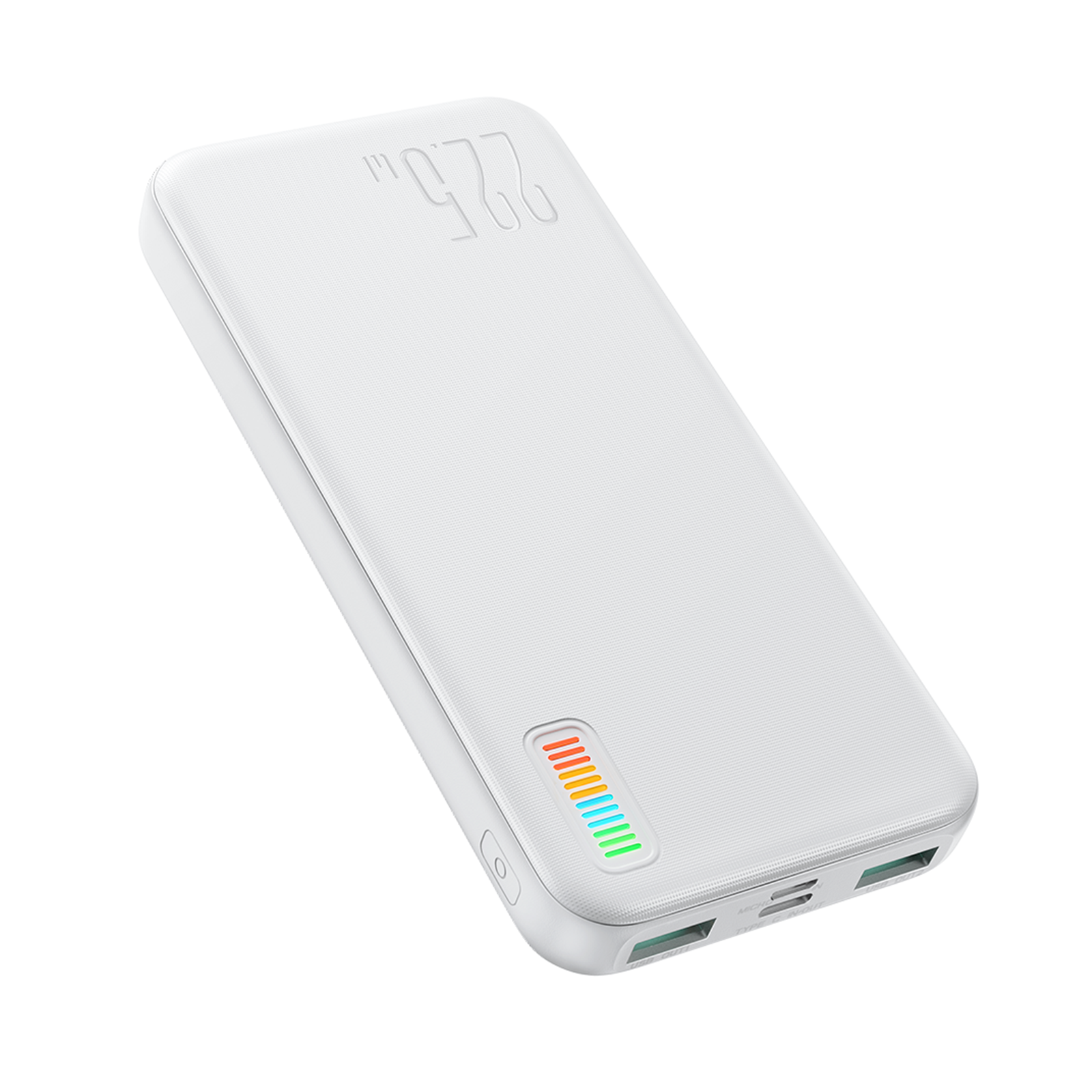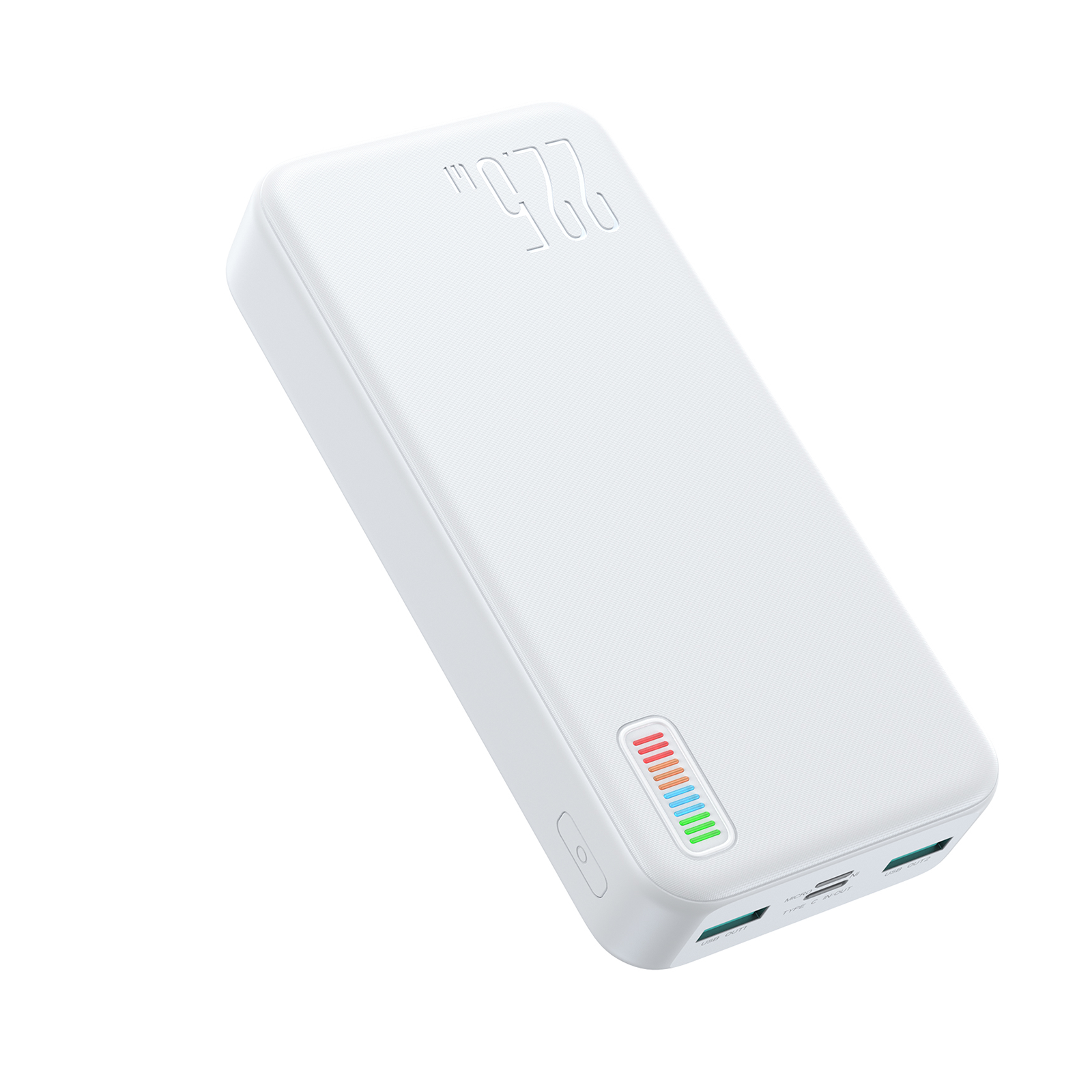# JR-QP194/195/196 Dazzling Series 22.5W Power Bank 10000mAh 20000mAh 30000mAh

Sale price\$25.88 USD
Colour:black
Capacity:10000mAh

## Features

1. 22.5W superfast charging, fully charge your phone in less time
2. 10000mAh capacity, ultra-slim body, easy to carry
(20000mAh/30000mAh large capacity, ultra-long battery life, make travelling easier)
3. 12 numbers of multi-colour LED indicators accurately indicate the remaining power
4. 2 input ports, three output ports, charge three devices simultaneously
5. Supports PD3.0, QC3.0, Samsung AFC and Huawei FCP, SCP fast charge protocols

## Parameters:

Model: JR-QP194
Battery Capacity: 10000mAh 3.7V (37Wh)
Rated Capacity: 5800mAh (5V=3A)
Energy Conversion Rate: ≥80%
Type-C Input: 5V=3A, 9V=2A, 12V=1.5A
Micro Input: 5V=2A, 9V=2A, 12V=1.5A
Type-C Output: 5V=3A, 9V=2.22A, 12V=1.67A
USB1/USB2 Output: 5V=3A, 9V=2A, 12V=1.5A, 10V=2.25A
Total output: 5V=3A (MAX)
Size: 146.5*70*16mm
Material: PC+ABS fireproof material
Net Weight: 237g

Model: JR-QP195
Battery Capacity: 20000mAh 3.7V (74Wh)
Rated Capacity: 12000mAh (5V=3A)
Energy Conversion Rate: ≥80%
Type-C Input: 5V=3A, 9V=2A, 12V=1.5A
Micro Input: 5V=2A, 9V=2A, 12V=1.5A
Type-C Output: 5V/3A, 9V=2.22A, 12V=1.67A
USB1/USB2 Output: 5V=3A, 9V=2A, 12V=1.5A, 10V=2.25A
Total output: 5V=3A (MAX)
Size: 146.5*70*29mm
Material: PC+ABS fireproof material
Net Weight: 425g

Model: JR-QP196
Battery Capacity: 30000mAh 3.7V (111Wh)
Rated Capacity: 18000mAh (5V=3A)
Energy Conversion Rate: ≥80%
Type-C Input: 5V=3A, 9V=2A, 12V=1.5A
Micro Input: 5V=2A, 9V=2A, 12V=1.5A
Type-C Output: 5V/3A, 9V=2.22A, 12V=1.67A
USB1/USB2 Output: 5V=3A, 9V=2A, 12V=1.5A, 10V=2.25A
Total output: 5V=3A (MAX)
Size: 146.5*70*41mm
Material: PC+ABS fireproof material
Net Weight: 613g